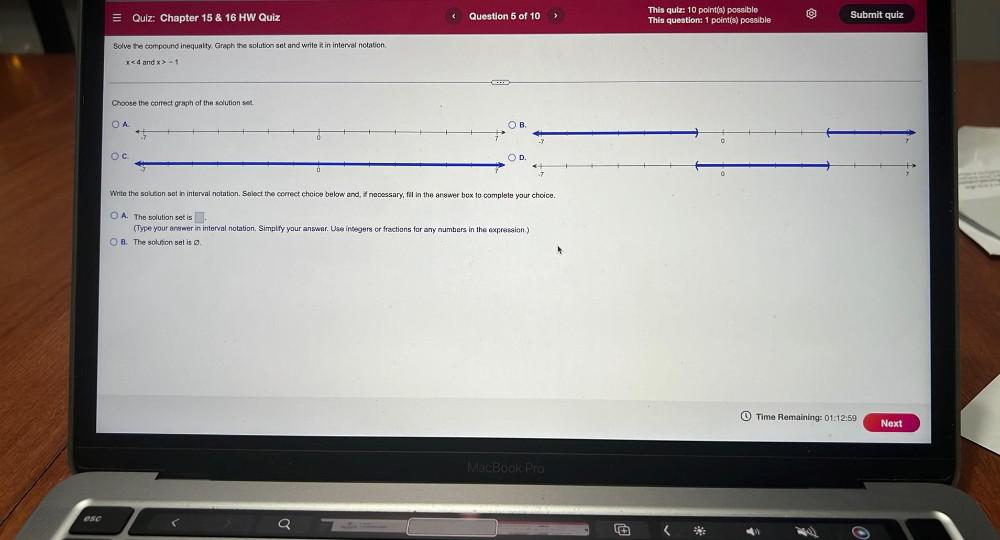Question:

# Solve the compound inequality. Graph the solution set and write it in interval notation. x < 4 and x > -1 Choose the correct graph of the solution set. Write the solution set in interval notation. SelSolve the compound inequality. Graph the solution set and write it in interval notation. x < 4 and x > -1 Choose the correct graph of the solution set. Write the solution set in interval notation. Select the correct choice below and, if necessary, fill in the answer box to complete your choice. (A) The solution set is ______. (Type your answer in interval notation. Simplify your answer. Use integers or fractions for any numbers in the expression.) (B) The solution set is Ø.